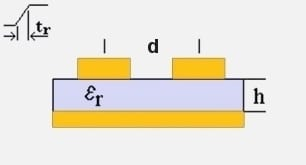Crosstalk

## Microstrip Crosstalk Calculator### Inputs

Source Rise Time
tr
ns
Source Voltage
v
Volts
Length of Parallel Traces
l
Substrate Height
h
Trace Pitch
d
Substrate Dielectric
εr

### Output ⊕

Cross Talk Coeff:
-0.158 dB
Coupled Voltage:
0.982 Volts

View Notes

#### Calculation Notes

Backward crosstalk is calculated by this tool, the other type is forward crosstalk which is less dominant. Crosstalk between traces is the (normally unwanted) coupled energy.

Factors that influence the crosstalk calculation include:-

• Source rise time (tr)
• Source voltage (v)
• Length of parallel traces (l)
• Substrate height (h)
• Trace pitch (d)
• Substrate dielectric (εr)

T_{RT} \approx 1.017\sqrt{\varepsilon _{r}\times 0.475+0.67}\times l \times 2  (round trip propagation time)

if

\frac{T_{RT}}{t_{r}}\leq 1

then

CT_{dB} \approx 20\log \left ( \frac{1}{1+\left ( \frac{d}{h} \right )^{2}}\times \frac{T_{RT}}{t_{r}} \right )

V_{crosstalk} \approx v\times \frac{1}{1+\left ( \frac{d}{h} \right )^{2}}\times \frac{T_{RT}}{t_{r}}

else

CT_{dB} \approx 20\log \left ( \frac{1}{1+\left ( \frac{d}{h} \right )^{2}}\right )

V_{crosstalk} \approx v\times \frac{1}{1+\left ( \frac{d}{h} \right )^{2}}

References:
• High-Speed Digital Design (ISBN 0-13-395724-1)
• Signal Integrity Issues and Printed Circuit Board Design (ISBN 0-13-335947-6)
• Douglas Brooks, "Crosstalk, Part 1: The Conversation We Wish Would Stop!", 1997

Disclaimer: The information and this tool are provided with no liability of any kind whatsoever, use at your own risk.